[Next] [Previous] [Up] [Top]

NUMERICAL METHOD FOR SPECIATION AND FORWARD MODELING

## Application to Initial Exchange Calculations

A limited set of equations is included in initial exchange calculations, that is, when the composition of an exchange assemblage is defined to be that which is in equilibrium with a specified solution composition. The Newton-Raphson equations for the initial exchange calculation are derived from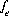,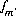,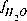, and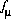, which are equations for mole balance for each exchanger, mole balance for each element or element valence state, activity of water, and ionic strength. For initial exchange calculations, the values of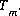include only the aqueous concentrations and the mole-balance equationsdo not contain terms for the contribution of the exchangers to the total element concentrations. The values calculated for all quantities related to the aqueous phase are the same as for the solution without the exchanger present. Essentially, only the values of the master unknowns of the exchange assemblage,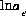, are adjusted to achieve mole balance for the exchanger mole-balance equations. Once mole balance is achieved, the composition of each the exchanger is known.

All equations for initial exchanger calculations are included as equality constraints in the solver. No equations are optimized and no inequality constraints are included.

An initial exchange calculation is performed only if the exchanger is defined to be in equilibrium with a specified solution. The distribution of species for this solution has already been calculated, either by an initial solution calculation or by a reaction or transport calculation. Thus, the initial estimates of all master unknowns related to the aqueous phase are set equal to the values from the previous distribution of species. The initial estimate of the master unknown for each exchanger is set equal to the number of moles of exchange sites for that exchanger.

For data input to PHREEQC, definition of the initial exchange calculation is made with the EXCHANGE keyword data block. (See Description of Data Input.)

User's Guide to PHREEQC - 07 MAY 96
[Next] [Previous] [Up] [Top]